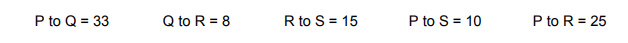# IMAT 2011 Q51 [Cross Over Values]

Cross over values (COV’s) can be considered as the relative distance between genes and are used to help construct chromosome maps. Four genes, called P, Q, R and S, are found on the same chromosome. Use the following COV’s to work out the sequence of the four genes.The sequence of the genes is

A. P Q R S

B. S P Q R

C. R S P Q

D. Q S R P

E. P S R Q

The answer to this question is E, where the sequence is P S R Q.

Do not get intimidated by this question, and approach it like a simple number scale where you have to assign letters where they belong, according to their relative distances, which have been provided to you in the question!

You will need to approach this question systematically to be able to reach the correct answer:

1. First, you will need to find the 2 ends of the sequence by looking at the letters that have the most relative distance from each other. In this case, P → Q is 33, where you can safely assume that these are the 2 ends. This is supported by the fact that the other two letters S and R are nearer to P than Q is. At this point, you will need to eliminate the choices that do not have P and Q at the ends, leaving you with only D and E as considerations.
2. Next, the challenge will be finding the relative distances of S and R to a reference point, which can be either P and Q since you are certain that they are the 2 ends of the sequence. Thus, look for relative distance to a reference letter that has BOTH S and R, which in this case, the question has given distances of S and R from P. Using Q in this question is not reliable, since its distances to all letters were not given.
3. If you look at the numbers, it will be clear that S is nearer to P than R is. This eliminates choice D, since that choice implies that R is nearer to P than S is, which is not correct.
4. This then leaves only one answer as correct, which is choice E, or the sequence P S R Q.